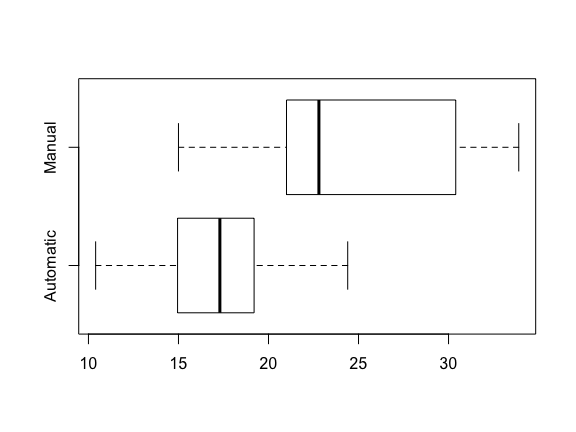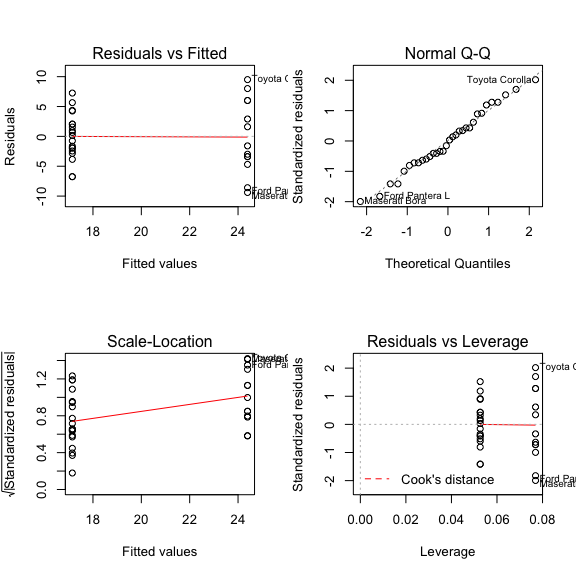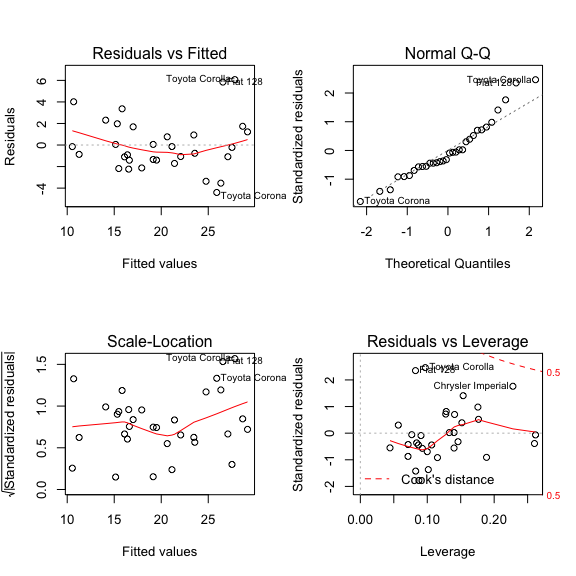## The Executive Summary

In this article we will examine some statistics relating to a set of cars to see if we can make predictions about fuel consumption (MPG). We will attempt to answer the following questions:

• Is an automatic or manual transmission better for MPG
• Quantify the MPG difference between automatic and manual transmissions

and then we shall perform some analysis to see if we can find better predictors of MPG.

## The Data

First a word about the data we shall use:

The data was extracted from the 1974 Motor Trend US magazine, and comprises fuel consumption and 10 aspects of automobile design and performance for 32 automobiles (1973-74 models).

And a brief description of the data:

Name Description
mpg Miles/(US) gallon
cyl Number of cylinders
disp Displacement (cu.in.)
hp Gross horsepower
drat Rear axle ratio
wt Weight (lb/1000)
qsec 1/4 mile time
vs V/S
am Transmission (0 = automatic, 1 = manual)
gear Number of forward gears
carb Number of carburetors

Now lets load the data, and convert the predictor of interest - am - into a factor:

    data(mtcars);
mtcars$am <- as.factor(mtcars$am);

Next lets plot the data to get a feel for the it. We’ll use a boxplot for this purpose:

    boxplot(mpg ~ am, mtcars, names = c('Automatic', 'Manual'), horizontal = TRUE);As you can see from the above boxplot, it would seem that cars with manual transmission out-perform cars with automatic transmission in terms of fuel consumption. In the next section we shall try to quantify this, and then see if we can build a better model for predicting fuel consumption.

## The Analysis

Lets fit a model to the data, with mpg as the dependent variable and am as the explanatory variable:

    fit1 <- lm(mpg ~ am, mtcars);
sum1 <- summary(fit1);
sum1;
##
## Call:
## lm(formula = mpg ~ am, data = mtcars)
##
## Residuals:
##     Min      1Q  Median      3Q     Max
## -9.3923 -3.0923 -0.2974  3.2439  9.5077
##
## Coefficients:
##             Estimate Std. Error t value Pr(>|t|)
## (Intercept)   17.147      1.125  15.247 1.13e-15 ***
## am1            7.245      1.764   4.106 0.000285 ***
## ---
## Signif. codes:  0 '***' 0.001 '**' 0.01 '*' 0.05 '.' 0.1 ' ' 1
##
## Residual standard error: 4.902 on 30 degrees of freedom
## Multiple R-squared:  0.3598, Adjusted R-squared:  0.3385
## F-statistic: 16.86 on 1 and 30 DF,  p-value: 0.000285

From the above summary we can see that the difference between the means of mpg for cars with automatic and manual transmissions is 7.2449393. This difference is quite significant, and is consistent with what we saw in the boxplot above. Lets plot some diagnostics to get a feel for how well the model is doing:

    par(mfrow = c(2, 2));
plot(fit1);The diagnostics look pretty decent, especially the normal q-q plot which measures the normality of the residuals. Lets construct a confidence interval for the difference in means:

    coef <- sum1$coefficients; ci1 <- coef[2,1] + c(-1, 1) * qt(.975, df = fit1$df) * coef[2,2];
ci1;
##   3.64151 10.84837

We are 95% confident that the difference in fuel consumption lies between 3.6415096 and 10.848369. Looking back at the values of $$R^2$$ (0.3597989) and adjusted $$R^2$$ (0.3384589) in the above model summary, it seems the variance in mpg is not well predicted by am. Perhaps we can do better:

    aov_full <- aov(mpg ~ ., mtcars[, 1:11]);
sum_full <- summary(aov_full);
sum_full;
##             Df Sum Sq Mean Sq F value   Pr(>F)
## cyl          1  817.7   817.7 116.425 5.03e-10 ***
## disp         1   37.6    37.6   5.353  0.03091 *
## hp           1    9.4     9.4   1.334  0.26103
## drat         1   16.5    16.5   2.345  0.14064
## wt           1   77.5    77.5  11.031  0.00324 **
## qsec         1    3.9     3.9   0.562  0.46166
## vs           1    0.1     0.1   0.018  0.89317
## am           1   14.5    14.5   2.061  0.16586
## gear         1    1.0     1.0   0.138  0.71365
## carb         1    0.4     0.4   0.058  0.81218
## Residuals   21  147.5     7.0
## ---
## Signif. codes:  0 '***' 0.001 '**' 0.01 '*' 0.05 '.' 0.1 ' ' 1

Looking at the output above, it seems that the three most relevant variables when it comes to predicting fuel consumption are cyl (Number of Cylinders), disp (Displacement), and wt (Weight). Lets fit a model using these variables and see what the resulting $$R^2$$ and adjusted $$R^2$$ values are:

    fit2 <- lm(mpg ~ cyl + disp + wt, mtcars);
sum2 <- summary(fit2);
sum2;
##
## Call:
## lm(formula = mpg ~ cyl + disp + wt, data = mtcars)
##
## Residuals:
##     Min      1Q  Median      3Q     Max
## -4.4035 -1.4028 -0.4955  1.3387  6.0722
##
## Coefficients:
##              Estimate Std. Error t value Pr(>|t|)
## (Intercept) 41.107678   2.842426  14.462 1.62e-14 ***
## cyl         -1.784944   0.607110  -2.940  0.00651 **
## disp         0.007473   0.011845   0.631  0.53322
## wt          -3.635677   1.040138  -3.495  0.00160 **
## ---
## Signif. codes:  0 '***' 0.001 '**' 0.01 '*' 0.05 '.' 0.1 ' ' 1
##
## Residual standard error: 2.595 on 28 degrees of freedom
## Multiple R-squared:  0.8326, Adjusted R-squared:  0.8147
## F-statistic: 46.42 on 3 and 28 DF,  p-value: 5.399e-11

The new value of $$R^2$$ (0.832607) and adjusted $$R^2$$ (0.8146721) are greater than the previous, suggesting the new model is a better predictor of fuel consumption. Once again, lets plot some diagnostics of the new model to get a feel for it’s performance:

    par(mfrow = c(2, 2));
plot(fit2);All the above plots look good - in particular the plot of residuals vs leverage would indicate there are no outliers with either high influence or leverage in the data. The residuals vs fitted plot looks reasonably uniform. The normal q-q plot would imply the residuals are normally distributed (except for at the right tail). All in all the model looks pretty good.

## The Conclusion

We have shown that manual transmissions are better than automatic in terms of fuel consumption. We quantified this difference by fitting a linear regression model and taking the relevant coefficient. We provided a 95% confidence interval for this difference. We analyzed the model with the aid of some diagnostic plots, and by considering the value of both $$R^2$$ and adjusted $$R^2$$, and decided to try for a better model. We analyzed the covariance of variables (ANOVA) and selected the three variables that were most related to mpg. We then constructed another model with these variables and saw that both $$R^2$$ and adjusted $$R^2$$ were greatly improved, and hence the model was a better predictor of fuel consumption.